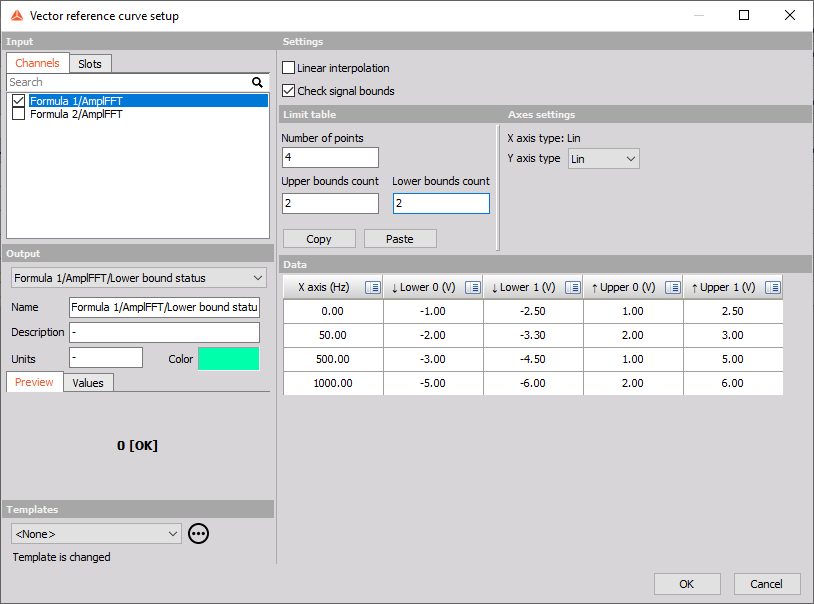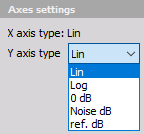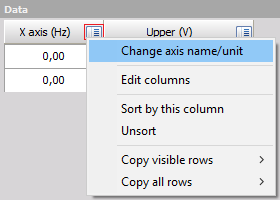# Vector reference curve

In the vector reference curve, we define the reference curves for the input channel, which is usually an array (FFT data, …).

When you press the Setup button on newly activated Vector reference curve line, the following setup screen window will open:## Settings

Vector reference curve math offers the following settings

1. Linear interpolation

With linear interpolation, the program will draw a direct line between the two neighborhood points. It will give a smoother transition between the points on the curve.

1. Check signal bounds

This will create an additional output channel which will output 1 when the input is higher than the reference curve and will output 0 when the input is below the reference curve

NOTE: When multiple bounds are added, the “Check signal bounds” function will output a value of 0 if the input signal is below all defined bounds. If the input signal is higher than the first bound, the function will output a value of 1. If the input signal is higher than the second bound, the function will output a value of 2, and so on for each additional bound that is defined

## Limit table

1. Number of points

Limit table points can be manually defined or by copy/paste from an external program.

1. Upper bounds count

Define a custom number of Upper bounds

1. Lower bound count

Define a custom number of Lower bounds

1. Copy/paste buttons

Wth copy paste buttons you can simply copy the values from the table to e.g. Excel, correct the values and paste the new values back to the Vector Ref Curve Math

NOTE: When multiple bounds are added and the “Check signal bounds” function is enabled, it’s important to ensure that the values of the bounds do not overlap. If there is an overlap between any of the bounds, the function will not be able to accurately determine which bound the input signal falls into and the check will be disabled.

## Axes settings

Axes settings can be selected from:• linear
• logarithmic
• 0 dB (dB reference will be set from the range of the first channel)
• Noise dB (the reference value is 20 uPa)
• ref. dB (user enters the reference value)

## Data

Rename the channels with the click on the icon in the top right corner and selecting the Change axis name/unit option.